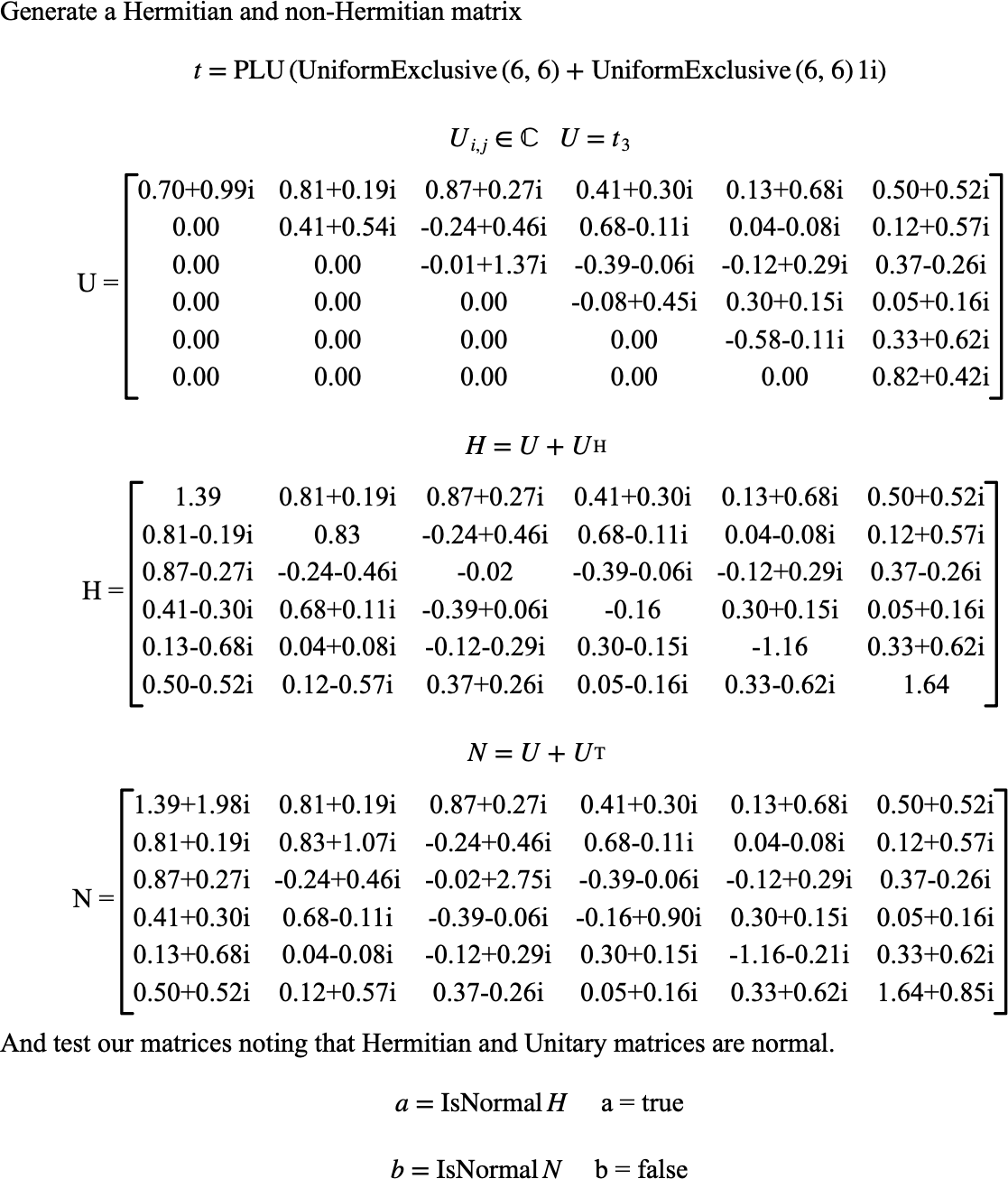# $$\text{IsNormal}$$¶

You can use the $$\text{IsNormal}$$ function to determine if a matrix is normal.

You can use the \isnormal backslash command to insert this function.

The following variants of this function are available:

• $$\text{boolean } \text{IsNormal} \left ( \text{<matrix>} \right )$$

• $$\text{boolean } \text{IsNormal} \left ( \text{<matrix>}, \text{<relative tolerance>} \right )$$

The $$\text{<matrix>}$$ parameter is the matrix to be tested. The optional $$\text{<relative tolerance>}$$ parameter specifies the tolerance to apply when comparing values. If not specified, the $$\text{IsNormal}$$ function will use a tolerance of $$1.0 \times 10^{-8}$$.

A matrix, $$M$$, is considered normal if $$A^H A = A A^H$$. The $$\text{isNormal}$$ function relaxes this constraint by performing the test within a given tolerance. The $$\text{IsNormal}$$ function considers a matrix to be normal if the matrix, $$M$$, is square and:

$M _ 1 = A^H A \;\;\;\;\; M _ 2 = A A^H$
$\left \vert M _ { 1,i,j } - M _ { 2,i,j } \right \vert \leq \frac{1}{2} \left \vert M _ { 1,i,j } + M _ { 2,i,j } \right \vert t _ {rel}$

For all $$1 \leq i \leq \text{nrows} \left ( M \right )$$ and $$1 \leq j \leq \text{ncols} \left ( M \right )$$ where $$t _ {rel}$$ is the relative tolerance. Note that this test is only applied if $$M _ { 1,i,j } \ne M _ { 2,i,j }$$.

Figure 159 shows the basic use of the $$\text{IsNormal}$$ function.Figure 159 Example Use Of The IsNormal Function